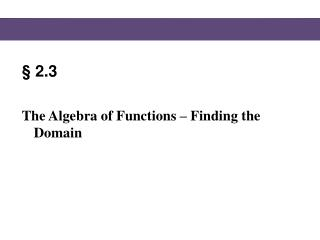DownloadDownload Presentation§ 2.3

§ 2.3

Download Presentation§ 2.3

- - - - - - - - - - - - - - - - - - - - - - - - - - - E N D - - - - - - - - - - - - - - - - - - - - - - - - - - -
Presentation Transcript

1. §2.3 The Algebra of Functions – Finding the Domain

2. Domain of a Function 117 Blitzer, Intermediate Algebra, 5e – Slide #2 Section 2.3

3. Domain of a Function 117 • Consider the function Because division by 0 is undefined (and not a real number), the denominator, x – 5, cannot be 0. Then x cannot be 5, and 5 is not in the domain of the function. Blitzer, Intermediate Algebra, 5e – Slide #3 Section 2.3

4. Domain of a Function 117 • Now consider the function: The equation tells us to take the square root of x – 7. Because only nonnegative numbers have square roots that are real numbers, the expression under the square root must be nonnegative. Then x must be greater than or equal to 7. Blitzer, Intermediate Algebra, 5e – Slide #4 Section 2.3

5. Domain of a Function 118 EXAMPLE Find the domain of the function: . SOLUTION Since the function f has no denominator or square root, there are no real numbers that when plugged into the function for x would cause the value of the function to yield something other than a real number. Therefore, the domain is: This is set notation and it is read: “the set of all x such that x is a real number.”. Using this notation, the rule stating the conditions for x follows the vertical bar which just means “such that.” Blitzer, Intermediate Algebra, 5e – Slide #5 Section 2.3

6. Domain of a Function 118 EXAMPLE Find the domain of the function: . SOLUTION The function has no square roots so we don’t have to worry about pursuing that avenue. However the function does have x in two different denominators. Therefore I do the following: Blitzer, Intermediate Algebra, 5e – Slide #6 Section 2.3

7. Domain of a Function 118 CONTINUED Set a denominator equal to zero Solve Set a denominator equal to zero Solve Therefore, a denominator of the function is equal to zero when x = 4 or x = -5. Then the domain is: Blitzer, Intermediate Algebra, 5e – Slide #7 Section 2.3

8. Domain of a Function 118 Check Point 1a Find the domain of the function: . SOLUTION Since the function f has no denominator or square root, there are no real numbers that when plugged into the function for x would cause the value of the function to yield something other than a real number. Therefore, the domain is: Blitzer, Intermediate Algebra, 5e – Slide #8 Section 2.3

9. Domain of a Function 117-118 Check Point 1b Find the domain of the function: . Because division by 0 is undefined (and not a real number), the denominator, x + 5, cannot be 0. Then x cannot be -5, and -5 is not in the domain of the function. Blitzer, Intermediate Algebra, 5e – Slide #9 Section 2.3

10. DONE